Question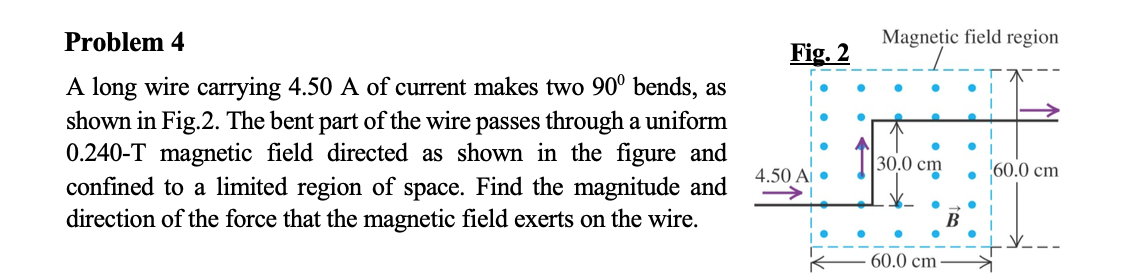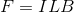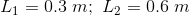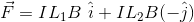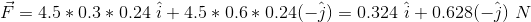#### Earn Coins

Coins can be redeemed for fabulous gifts.

Similar Homework Help Questions
• ### A long wire carrying 4.50 A of current makes two 90 degree bends, as shown in....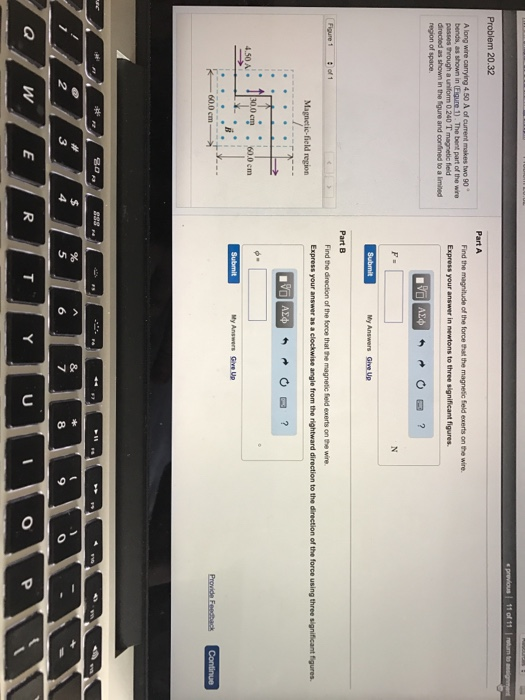A long wire carrying 4.50 A of current makes two 90 degree bends, as shown in. The best part of the wire passes through a uniform 0.240 t magnetic field directed as shown in the figure and confined to a limited of space. Part A Find the magnitude of the force that the magnetic field exerts on the wire Part B Find the direction of the force that the magnetic field exerts on the wire.

• ### A long wire carrying 4.50 A of current makes two 90 bends, as shown in the...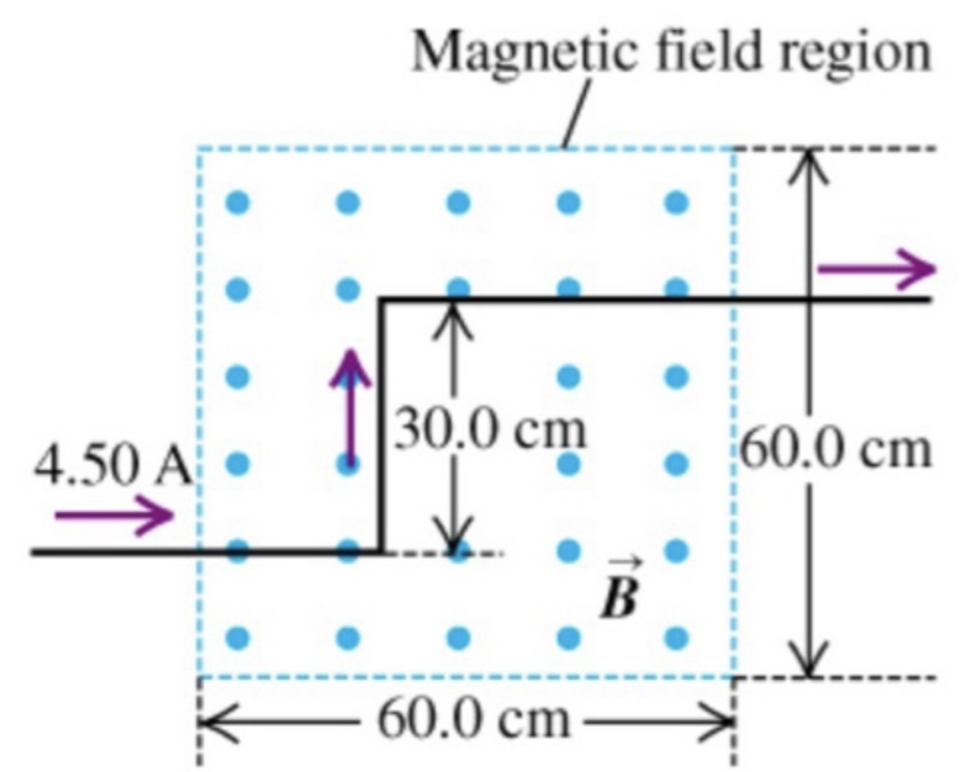A long wire carrying 4.50 A of current makes two 90° bends, as shown in the figureThe bent part of the wire passes through a uniform 0.238 T magnetic field directed as shown in the figure and confined to a limited region of space.Part AFind the magnitude of the force that the magnetic field experts on the the wire.Part BFind the direction of the force that the magnetic field experts on the the wire.

• ### A long wire carrying 6.50 A of current makes two bends, as shown in the figure.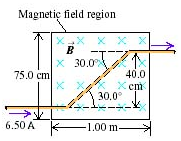A long wire carrying 6.50 A of current makes two bends, as shown in the figure. The bent part of the wire passes through a uniform 0.280 T magnetic field directed as shown in the figure and confined to a limited region of space.A) Find the magnitude of the force that the magnetic field exerts on the wire.B) Find the direction of the force that the magnetic field exerts on the wire.

• ### ​A long wire carrying 6.50 A of currents makes two bends, as shown in the figure on the right.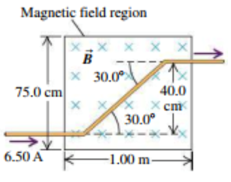A long wire carrying 6.50 A of currents makes two bends, as shown in the figure on the right. The bent part of the wire passes through a uniform 0.280-T magnetic field directed as shown and confined to a limited region of space. a. What are the magnitude and direction of the force exerted on the wire by the magnetic field?b. Now assume that the magnetic field extends 1.00 m to the left and to the right of the region shown...

• ### A long wire carrying a 6.00A current reverses direction by means of two right-angle bends, as...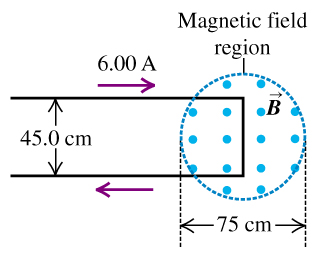A long wire carrying a 6.00A current reverses direction by means of two right-angle bends, as shown in the figure (Figure 1) . The part of the wire where the bend occurs is in a magnetic field of 0.666T confined to the circular region of diameter 75cm, as shown. A) Find the direction of the net force that the magnetic field exerts on this wire. B) Find the magnitude of the net force that the magnetic field exerts on this...

• ### An infinitely long wire carrying a current of 4.5 A is bent at the origin and...

An infinitely long wire carrying a current of 4.5 A is bent at the origin and extends in both the y-direction and x-direction. (In other words, the wire makes a 90 degree angle with the x axis and y axis and has a bend at the origin) Find the magnetic field at the point x = 3 cm and y = 2 cm.

• ### An infinitely long wire carrying a current of 4.5 A is bent at the origin and...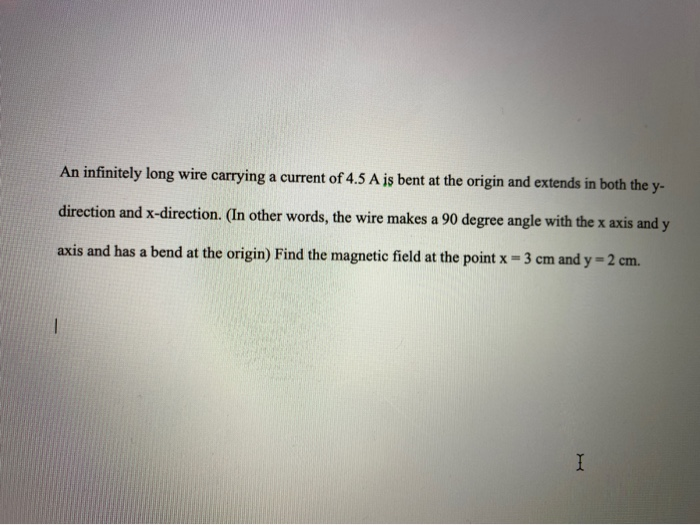An infinitely long wire carrying a current of 4.5 A is bent at the origin and extends in both the y- direction and x-direction. In other words, the wire makes a 90 degree angle with the x axis and y axis and has a bend at the origin) Find the magnetic field at the point x = 3 cm and y = 2 cm.

• ### problem #4 #7 4. Problem A long wire is bent to form a rectangular section and is placed in a region with a uniform...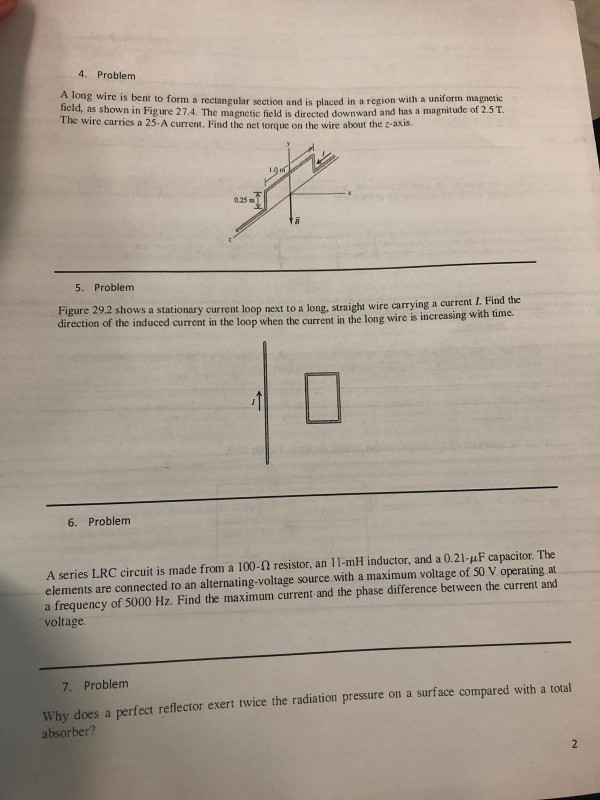problem #4 #7 4. Problem A long wire is bent to form a rectangular section and is placed in a region with a uniform magnetic id, as shown in Fig ure 27.4. The magnetic field is directed downward and has a magnitude of 2.5T. The wire carries a 25- A current. Find the net torque on the wire about the z-axis. 025m 5. Problem Figure 29.2 shows a stationary current loop next to a long, straight wire carrying a current...

• ### Wire Ring . A uniform magnetic field is confined to a cylindrical volume of radius 8...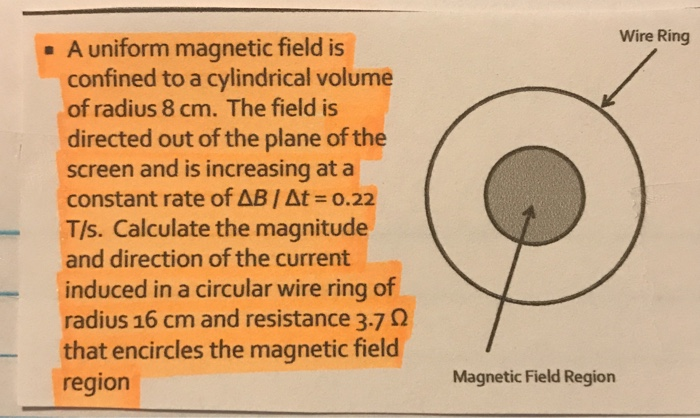Wire Ring . A uniform magnetic field is confined to a cylindrical volume of radius 8 cm. The field is directed out of the plane of the screen and is increasing at a constant rate of AB At = 0.22 T/s. Calculate the magnitude and direction of the current induced in a circular wire ring of radius 16 cm and resistance 3.7 12 that encircles the magnetic field region Magnetic Field Region